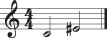##### Music Theory For Dummies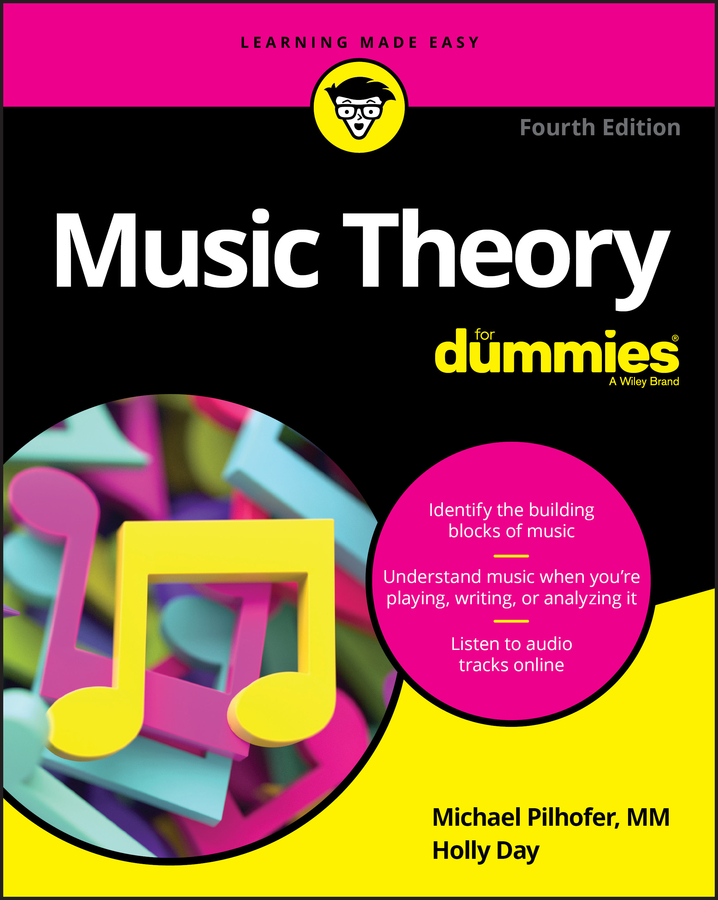Music theory can seem a little daunting. Just the phrase compound interval can sound a bit complicated and scary — maybe because of the word compound itself. Yet, the truth of the matter is that creating compound intervals won’t be completely foreign if you’re familiar with any other interval.

What distinguishes a compound interval from other intervals is that a compound interval is not confined to one octave the way a simple interval is. A compound interval can be spread out over several octaves, although the notes of the chords most often just come from two neighboring octaves.

## Creating a compound interval

Just how do you go about building a compound interval? You have two options:

• Count the interval between notes by half steps, as with the tenth.

• Take your compound interval, put both notes in the same octave, figure out the number size of that interval, and then add seven to the number size of the resulting interval.

Here’s an example of how to create a compound interval using this second method: Take a look at the simple intervals in the C major scale. Now, turn each of those figures into a compound interval by adding an octave to the second note in each example.

When you add an octave to a major second interval (M2), it becomes a major ninth (M9). When you add an octave to a major third (M3), it becomes a major tenth (M10). A perfect fourth (P4) becomes a perfect eleventh (P11), while a perfect fifth (P5) becomes a perfect twelfth (P12). The quality of the interval remains the same — all that’s been done is an extra octave’s been added to the quantity (+7).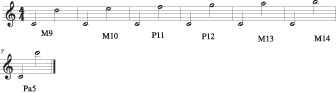The first note in the simple intervals is exactly the same as the first note in the compound intervals. The second note in the compound interval is the same note as the second note in the simple interval, just one octave higher. The quality of each interval remains the same, but the size number has increased by one octave, or the original number plus 7.

If you were to increase the distance between the notes by yet another full octave, you would simply add 7 to the size number again to reflect the interval size: Major 16, Major 17, Perfect 18, Perfect 19, Major 20, Major 21, and Perfect 22.

## Returning a compound to its simple state

Finding the quantity and quality of a compound interval is very similar to the process you can use to build a compound interval. Just reduce the compound interval to a simple interval by putting both notes in the same octave, either by moving the first note up or the second note down.

Here’s how it works: You have a compound interval of unknown quantity and quality. If you don’t mind counting out all the lines and spaces (10) between the two notes (C and E sharp) to find the quantity, then you don’t have to reduce the interval to a simple interval. However, most people find it easier to figure out the quantity and quality of an interval if the notes are closer together on the staff.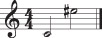Now, reduce the compound interval to a simple interval by simply bringing the two notes closer together so that they’re in the same octave. You can either bring the first note up an octave, or the second note down an octave.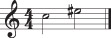In its reduced state, the quantity of this interval pictured is a third. When you take a third and raise the upper note a half step or lower the bottom note a half step, the interval becomes an augmented third (A3). Add an octave, and the compound interval’s quantity and quality is an A10, or the simple interval quantity and quality, plus 7. Quality has no bearing on octave displacement — only the quantity changes.